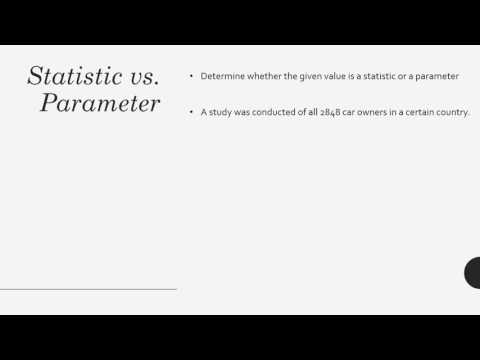### Main Difference

People often intermix with the terms like statistic and parameter, which are widely used in the statistics language. Before hurling to these concepts, one needs to know more about the statistics, which holds both the concepts, parameter and statistic into it. The statistics is the branch of mathematics which deals with the collection, analysis, interpretation, and presentation of masses of numerical data. The terms statistic and parameter can be differentiated as the parameter describes or denotes the entire population, whereas statistic describes or denotes a fraction or sample from the whole. With this definition, we can derive that in statistic we do an analysis of the large population, on the other hand, in parameter we usually analyze the small populations as each of them can be measured in it.

### Comparison Chart

 Statistic Parameter Definition The statistic is the numerical value we get after obtaining the sample data from the entire population. The parameter is the numerical value we get in statistics after evaluating or all analyzing all the elements present in the entire population. Population In statistic we came across the large population as just samples out the whole are analyzed in this case. In parameter we came across the small population, in which each can be measured. Accuracy The statistic is variable which totally relies on the samples taken from the population. The parameter is the fixed and more accurate value as we examine the entire population in it.

### What is Statistic?

The statistic is the numerical value we get after obtaining the sample data from the entire population. In this we analyze large values by taking samples out of them. The samples we obtain and use represent the entire population. The sample taken from the entire population for knowing the statistics is known as the fraction of the population as in this the data is collected from some elements present in the population, but it stands for the entire population. In some cases while making the results about certain population we take multiple samples so we can get the better representation of the whole population. The results taken from each of the samples in this case are quite different to each other. When we have to figure out that whether it is that statistic or the parameter of the population, we need to follow a simple rule. When there is the large population in which every element can’t be measured individually it is the statistic. For instance, when we say that 60% of US residents disagree with the Trump’s latest ban on Muslims. That means we have got the results through statistics as each and every individual’s opinion is not taken over the matter, the different samples taken from the entire population are analyzed in the case.

### What is Parameter?

The parameter is the numerical value we get in statistics after evaluating or all analyzing all the elements present in the entire population. The parameter is the fixed and more accurate value as compared to the statistic as in this entire population is surveyed. At the very same time, the parameter is the time-taking process as it is the descriptive measure of the entire population so that means they cannot be directly calculated on the basis of samples within the population. The samples analyzed in this are not based on estimation, and the values are deduced comprehensively from each and every of the samples that sum up to the entire population. Generally in the parameter we deal with the small population so that each and every of the element is analyzed. Although, in census we measure using the process of the parameter. Even though in census sometimes there is large population, but each and every of them is surveyed. That is why the census is time-taking data analyzing processes.

### Statistic vs. Parameter

• The parameter describes or denotes the entire population, whereas statistic describes or denotes a fraction or sample from the whole.
• In parameter we came across the small population, in which each can be measured. On the other hand, in statistic we came across the large population as just samples out the whole are analyzed in this case.
• The statistic is variable which totally relies on the samples taken from the population while the parameter is the fixed and more accurate value as we examine the entire population in it.